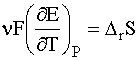### Re: How can some aqueous ions be listed as having a negative entropy?

Date: Fri May 7 15:42:07 1999
Posted By: Dan Berger, Faculty Chemistry/Science, Bluffton College
Area of science: Chemistry
ID: 925416664.Ch
Message:

How can some aqueous ions be listed as having a negative entropy?

I thought that the smallest entropy assigned was zero (for a perfect crystal at zero Kelvin). How can some aqueous ions be assigned a negative value in thermodynamic tables?

There are two levels of answer to your question. The first level is this, taken from PW Atkins, Physical Chemistry, 3d Ed: Solution reaction entropies can be obtained electrochemically via the relationshipwhere n is the number of electrons involved in the reaction, F is Faraday's constant and E is the electromotive force. The subscript "r" indicates that the entropy is for a reaction, rather than an entropy of formation.

Furthermore, the standard partial molar entropy of the aquated proton is defined as zero:

Sø(H+, aq) = 0

Relative to this, many ions have negative solution entropies.

Now solution is very different from the solid state; we are really comparing apples to oranges here. Even so, we can make some hand-waving statements on the order of "well, the apple is red and the orange is orange, and they have different detailed shapes."

A perfect crystal is in a highly ordered state, but its component ions are not imposing order on their surroundings, so to speak. However, in solution the ions are solvated. They are therefore imposing a great deal of order on their surroundings, compared to the random arrangements of water molecules in pure water. You have gone from pure water plus a perfect crystal, to a series of ordered aggregates of the form ion· nH2O.

And remember that solvated H+ is assigned an entropy of zero. H+ is the smallest ion and so can't surround itself with as many water molecules as, say, Na+.

Current Queue | Current Queue for Chemistry | Chemistry archives